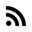# Details of C that every not so noob forgets

I already wrote a post about Details of C that every noob forgets. In this post I will write about more advanced details. I hope this will improve your understand of the obscure C. By the way, for the a complete review I recommend to read Expert C Programming: Deep C Secrets by Peter van der Linden or my notes.

# WTF? `const` is not constant

The keyword `const` doesn’t mean constant expression , it only gives the status of read-only.

``````const int two=2;

switch (i) {
/*case const-expr: statements*/
case 1: printf("case 1 \n");
case two: printf("case 2 \n");
**error** ^^^ integral constant expression expected
case 3: printf("case 3 \n");
default: ; }
``````

# Typedef vs macros

Even though they might look the same, these guys are totally different.

``````#define type1 int

typedef  int type2;

int main(){

type1 a; // works
type2 b; // works

unsigned type1 c; // works
unsigned type2 d; // illegal
}
``````

`typedef` defines a new data type, while `#define` is just a replacement. For example `typedef int type2;` means that `type2` is int, whereas `#define type1 int` means that `type1` will be replaced by `int` every time it appears in the code. Thus, you can even to the following:

``````#define type1 int
typedef  type1 type2;
``````

Again `#define` does not define a new data type.

``````#define int_ptr int *
int_ptr a,b;
/*
this means:
int *a, b;
which is different from
int *a, *b;
*/
``````

# Do not self-deSTRUCT

I used to make this mistake when I was learning C. Be careful when using `typedef` with `struct`.

``````// struct with foo as a tag
struct foo{
int a;
char b;
};

// foo1 is a struct foo
typedef struct foo{
int a;
char b;
} foo1;
``````

Check the following statements:

``````foo a;  // illegal
// there is not 'foo' type
struct foo a; // good
foo1 a; // good
struct foo1 a; // illegal
// foo1 is already a data type
``````

# EPIC BATTLE: arrays vs pointers

## Accessing data from array is different if we use pointers.

``````char a = "abcd";
c = a;

/*
in symtab: a is on 0x4000
a = | a | b | c | d | '\0' |
*/
``````

If we want to access the third element `a`

1. 0x4000 + 2 , directly!!
2. get value from 0x4002
``````0x0000000000400509 main+19 movzbl -0x1e(%rbp),%eax
0x000000000040050d main+23 mov    %al,-0x1(%rbp)
``````

In case of pointers:

``````char *p = a;
c = *(p+2);
``````

To access the third element:

2. p+2
3. get value from that address
``````0x000000000040052d main+55 mov    -0x10(%rbp),%rax
0x0000000000400531 main+59 movzbl 0x2(%rax),%eax
0x0000000000400535 main+63 mov    %al,-0x1(%rbp)
``````

## The array definition will allocate N bytes, whereas a pointer definition will only allocate a word size.

``````int m;
/* sizeof(m) = 12 */
int *p;
/* sizeof(p) = 8 (CPU of 64 bits)*/
``````

Another example:

``````char a[] = "hallo";
char *b  = "hallo";
``````

`a[]` is an array that holds `{'h','a','l','l','o','\0'}`, and it can be modified. `*b` is a pointer to a read-only section that holds `"hallo"`.

One last example:

``````int main(){
// an array
char a[] = "hola";

// a pointer
char *b;

// a pointer to read-only memory
char *c = "hallo";

// b can point to a or c
b = c;
b = a;

// c can point to a or c
c = a;
c = b;

// but a cannot point to a or c,
// because it is not a FUCKING POINTER!!!!!

a = b;
// error: assignment to expression with array type   a = b;

}
``````

## When an array is a pointer

Declarations themselves can be further split into three cases:

• declaration of an external array `extern char a[];`. `a` cannot be rewritten as a pointer.
• definition of an array (remember, a definition is just a special case of a declaration; it allocates space and may provide an initial value). `char a;`. `a` cannot be rewritten as a pointer.
• declaration of a function parameter `int function ( char a[]); `. `a` is rewritten as a pointer whether you like it or not.
``````void my_function_1( int a  ) { ; }
void my_function_2( int a [] ) { ; }
void my_function_3( int (*a) ) { ; }

int main(){
int arr;

my_function_1( arr );
my_function_2( arr );
my_function_3( arr );

int (*p)  = arr;

my_function_1( p );
my_function_2( p );
my_function_3( p );

int (*q) = &arr;

my_function_1( *q );
my_function_2( *q );
my_function_3( *q );
}
``````

In summary, the rule array name is rewritten as a pointer argument isn’t recursive. An array of arrays is rewritten as a pointer to arrays NOT as a pointer to pointer.

Thus,

• array of array `char a` can be rewritten as `char (*a)` pointer to array
• array of pointers `char *a` can be rewritten as `char **a` pointer to pointer
• pointer to array `char (*a)` is `char (*a)` pointer to array
• pointer to pointer `char **a` is `char **a` pointer to pointer

The very common case is the `main` function:

``````int main(int argc, char **argv){}

int main(int argc, char *argv[]){}
``````

# Just for fun

``````main() { printf(&unix["\021%six\012\0"],(unix)["have"]+"fun"-0x60);}`
``````

David Korn wrote this one-liner and won the iocc contest in 1987

Try to understand yourself the code, there are some extra info you might need:

• `unix` is a predefined macro with value 1 `#define unix 1`
• `a[i]` is resolved by the compiler as `*(a+i)`
• `a[i][j]` is resolved by the compiler as `*( *(a+i) + j)`

# Last words

C is a very simple language if you compare to python or even c++, and still very powerful. I hope you enjoy all the information provided here. Let me know if you have some questions.

Have fun!